## Determination of h Parameters from Static Characteristics:

Functional relationships for the CE configuration of total instantaneous collector current and base voltage in terms of two variables (base current and collector voltage) are given from earlier equations respectively. Such functional relationships are represented by families of characteristic curves of transistors, already described. Two families of curves are usually specified for transistors. The output characteristic curves depict the relationship between the output current and voltage, with input current as the parameter. As we know the typical output characteristic curves for the common-base, common-emitter and common-collector configurations of a transistor. The input characteristic curves give the relationship between input voltage and current with output voltage as the parameter. Typical input characteristic curves for the common-base, common-emitter and common-collector configurations of a transistor are already illustrated respectively. Determination of h Parameters from Static Characteristics is developed graphically from the input and output characteristics of a transistor for a particular configuration.

### Determination of Hybrid Parameters hfe and hoe

For a common-emitter configuration, the output characteristic curves are reproduced in Fig. 11.9. In this figure let us consider the curve for iB = IB = 60 μA. At a point Q on this curve, the quiescent collector current and collector voltage are IC and VC respectively. If a vertical straight line is drawn through point Q intersecting curves for iB1 = 40 μA and iB2 = 80 μA at points Q1 and Q2 respectively, the corresponding collector currents will be ic1 and ic2 respectively,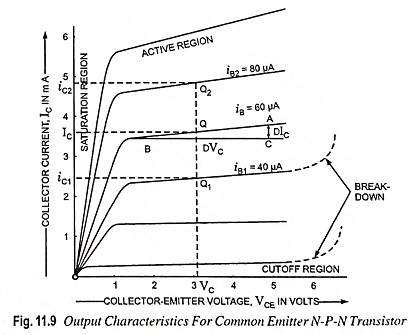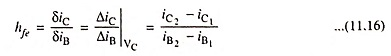and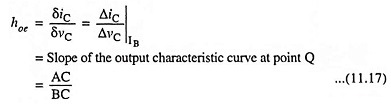Thus the value of hoe at point Q is given by the slope of the output characteristic curve at that point. Thus slope can be determined by drawing a line tangential to the characteristic curve at the point Q. The slope can also be determined by drawing an incremental triangle ABC about point Q and noting the values of AC and BC.

The parameter hfe is the most important transistor small-signal parameter. This common-emitter current transfer ratio, or CE alpha, is also written αe or β′, and called the small-signal beta of the transistor.

### Determination of Hybrid Parameters hre and hie

For a common-emitter configuration, the input characteristics are shown in Fig. 11.10. In this figure let us consider the curve for vC = VC. At a point Q on this curve, the quiescent base voltage and base current are VB and IB respectively. If a vertical straight line is drawn through this point Q intersecting curves for vC1 and vC2 at points Q1 and Q2 respectively, the corresponding values of base voltages will be vB1 and vB2 respectively.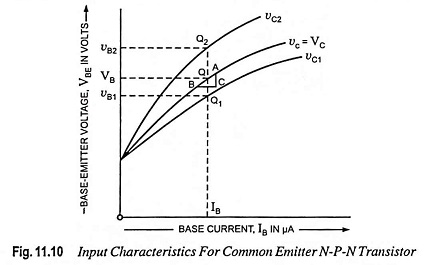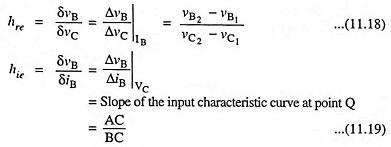Since hre is of the order of 10-4 so ΔvB≪ΔvC and hence the above method is not accurate in practice, though it is correct in principle.

Slope of the input characteristic curve giving the value of hie can be determined by either drawing a straight tangential line to the input characteristic curve at point Q or by drawing an incremental triangle ABC about point Q and noting the values of AC and BC.

The above procedure explained for the Determination of h Parameters from Static Characteristics of the common-emitter can also be used for determination of the common-base and common-collector h parameters from the appropriate input and output characteristics.

Scroll to Top